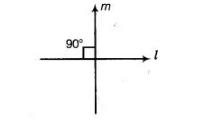# If one of the angles formed by two`
Question:

If one of the angles formed by two intersecting lines is a right angle, what can you say about the other three angles? Give reason for your answer.

Solution:

Let two intersecting lines l and m makes a one right angle, then it means that lines I and m are perpendicular each other. By using linear pair axiom aniom, other three angles will be a right angle.